Next: Filter Circuits Up: Alternating Current Circuits Previous: Single-Term Approximations of H

# Problems

1. Consider the following circuit: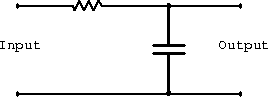1. What is the impedance of the circuit?
2. For the input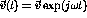, what current flows through the capacitor?
3. What is the phase difference between the voltage across the capacitor (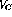) and the applied voltage? Be sure to specify whetherleads or follows the applied voltage.
2. Consider a circuit consisting of an inductor (L), a capacitor (C) and a resistor (R) in series with a voltage source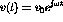. Let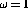krad/s, R=1 k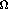, L=1 H and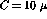F.
1. What is the magnitude of the current through the circuit?
2. What is the phase of the current relative to the source voltage?
3. What is the magnitude of the voltage drop across the inductor?
4. What is the phase of the voltage across the inductor relative to the source voltage?
5. At what frequency is the amplitude of the voltage across the resistor largest?
3. Derive the transfer function for the four-terminal network shown below.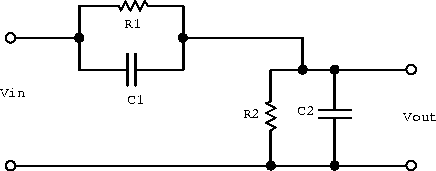Doug Gingrich
Tue Jul 13 16:55:15 EDT 1999